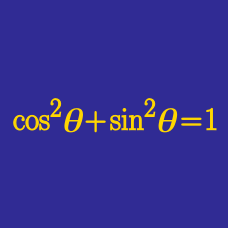Geometry

# Trigonometric Periodicity Identities

Which of the following is equal to $$3\cos(11\pi+x)?$$

Which of the following is equal to $$2\tan\left(x-\frac{5}{2}\pi\right)?$$

Which of the following is equal to $\sin^2(5\pi+x)+\cos^2(-4\pi-x)+\tan^2\left(\frac{5}{2}\pi+x\right)$ for a random $$x?$$

Find the value of $$\sin\left(-\frac{5}{3}\pi\right)+\cos\left(\frac{59}{6}\pi\right)+\tan\left(\frac{31}{3}\pi\right).$$

Which of the following is equal to $$\sin\left(\frac{39}{2}\pi+3x\right)?$$

×